# Kerala Syllabus 9th Standard Physics Solutions Chapter 5 Work, Energy and Power

You can Download The Last Leaf (Story) Questions and Answers, Summary, Activity, Notes, Kerala Syllabus 9th Standard Physics Solutions Part 2 Chapter 5 help you to revise complete Syllabus and score more marks in your examinations.

## Kerala State State Syllabus 9th Standard Physics Solutions Chapter 5 Work, Energy and Power

A The Trio (Story) Textual Questions and AnswersHss Live Guru 9th Physics Kerala Syllabus Chapter 5 Question 1.
Observe figure try to write down the activities shown in them.

1. A man pushes a trolly.
2. Batting of a cricket ball.
3. Pushing a wall.

Kerala Syllabus 9th Standard Physics Notes Chapter 5 Question 2.
Write down more activities familiar to you

1. A man carrying a load
2. Throwing a ball
3. Lifting the bag on to the shoulder
4. Pushing a car into motion

Hsslive Guru 9th Physics Kerala Syllabus Chapter 5 Question 3.
You have understood that a force is to be applied on a body to do an activity. Find out the source of applied force for every activity and note them down in the table.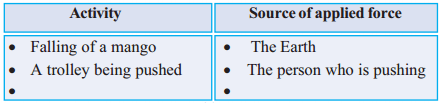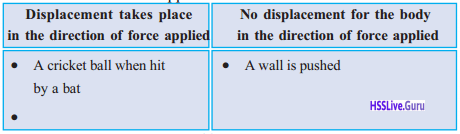Activity Source of applied force Falling of a mango The earth A trolley being pushed The person pushing Batting of a cricket ball Batsman Pushing a wall The person pushing Lifting a load The person lifting

Objects undergo displacement only when the force is applied on it them.

 Displacement takes place in the direction of force applied No displacement for the body in the direction of force applied 1. A cricket ball when hit by a bat 1. A wall is pushed 2. A trolley is being pulled. 2. A car is pushed by sitting inside the car 3. Climbing a ladder with a load on head. 4. Falling of a mango from mango tree.

Work :

Work is said to be done only when a body under¬goes displacement in the direction of the applied force.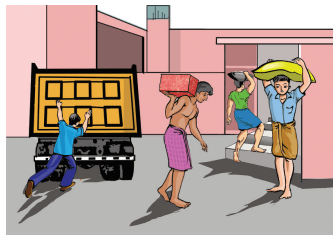work energy and power Question 4. Observe figure and write down the situation where work is said to be done

Kerala Syllabus 9th Physics Notes Chapter 5 Question 5.
A boy pushed an object of mass 30Kg horizontally across the floor through 50m. Another boy pushed an object of mass 50kg across the same floor through 50m. Both of them gave the same speed for moving the objects.
a) Who applied greater force here?
b) In which case was the work greater?
c) Write down a factor influencing work.
a) Second boy applied greater force
b) More work is done in the second situation
c) The Factor influencing work is force.

Learn Mass Transfer MCQ questions & answers are available for a Chemical Engineering students.

Hss Live Guru Physics 9th Kerala Syllabus Chapter 5 Question 6.
A boy pushed an object of mass 30kg across a horizontal floor through 20m. Another boy pushed the same body through 30m on the same floor with the same speed.
a) Who pushed a greater distance here?
b) What about the force applied
c) Who did the greater work?
d) Which is the factor influencing work here?
a) Second boy
b) Force is same
c) Second boy done greater work
d) The Factor influencing work is displacement

The factor affecting work done are force (F) and displacement (S)
Equation for calculating work done Work W = Fs
Unit of work is Joule (J), Kilojoule (KJ)
1KJ = 1000 J

If a force of F newton is applied continuously on a body and the body undergoes a displacement of s metre in the direction of the force, then the work done by the applied force is W = Fs

Get the free “Nuclear Equation Calculator” widget for your website, blog, WordPress, Blogger, or iGoogle.

Physics Notes For Class 9 Kerala Syllabus Chapter 5 Question 7.
When a force of 10N is applied continuously on a body it undergoes a displacement of 2m find out the magnitude of the work done?
F = 10N, s = 2m
Work W= Fs = 10 × 2 = 20 Nm
Look at the FigureHsslive Guru Physics Class 9 Kerala Syllabus Chapter 5 Question 8.
A body of mass m kg is placed on a table. What are the forces experienced by this body?
Weight of the body applies downwards and the table applies an equal force upwards.

Hss Live 9th Physics Kerala Syllabus Chapter 5 Question 9
In which directions do these forces act?
Forces acting on both directions, upward direction and downward direction.

9th Physics Notes Kerala Syllabus Chapter 5 Question 10.
A book of mass 100g is raised to the top of a table of height 1 m. Find the magnitude of the work done by the force applied against the gravitational force(g = 10m/s2)
m = 100g = 0.1kg
g = 10m/s2
h = 1m
W = mgh
= 0.1 × 10 × 1 = 1J
1 J is the amount of work done to raise a body of mass 100 g through a height of 1m.

Kerala Syllabus 9th Standard Physics Solutions Chapter 5 Question 11.
If a force of 50N is applied on a body and it under¬goes a displacement of 2m in the direction of the force, calculate the amount of work done.
F = 50N
s = 2m
W = Fs = 50 x 2 = 100J

Hss Live Guru 9 Physics Kerala Syllabus Chapter 5 Question 12.
a) If a force of 200N is applied on a table of mass 50kg, it undergoes a displacement of 0.5m in the direction of force. Calculate the amount of work done.
b) If the same table is raised by 3 m, what would be the work done against the gravitational force?
a) F= 200N, s = 0.5m
W = Fs = 200 x 0.5 = 100J
b) m = 50 kg, g = 10m/s2
h = 3 m
W = mgh = 50 x 10 x 3 = 1500J
Observe FigureLet a body mass m be pulled by a force F. If the body has a displacement s in the direction of the force, then the work done by the force F, Wf = Fs Here the displacement produced is in the direction of the force itself.

Kerala Syllabus Class 9 Physics Solutions Chapter 5 Question 13.
Write whether this work is negative or positive.
Work is positive

9th Class Physics Notes Kerala Syllabus Chapter 5 Question 14.
The displacement is opposite to the frictional force, is the work done by the frictional force positive or negative
If the displacement is in opposite direction work done by frictional force is negative.

Class 9 Physics Kerala Syllabus Chapter 5 Question 15.
In which direction is the force of gravity on the body?
Direction of the gravitational force will be in the downward direction

9th Standard Physics Notes Kerala Syllabus Chapter 5 Question 16.
Is there a displacement for the body in the direction of the gravitational force?
No displacement occurs in the direction of the gravitational force.
When a body on a floor is pulled and if it is displaced in the direction of the applied force, the work done by the applied force will be positive and the work done by the frictional force exerted by the floor will be negative.

Energy

Question 17.
What is the work to be done to raise a body of mass m kg through h meter?
Physics chapter 5 work and energy Answer:
w = mgh
Energy is the capacity to do work. Unit is Joule (J)

work energy book Question 18. In daily life, we use different forms of energy for various activities. List the forms of energy familiar to you

1. Mechanical energy
2. Heat energy
3. Electrical energy
4. Chemical energy
5. Light energy
6. Nuclear energy

There are two type of Mechanical energy Kinetic energy and potential energy

Kinetic Energy

Pulling the toy car backwards a little and allow it to hit the plastic ballQuestion 19.
What happened to the ball when the moving car hit it?
Ball moves forward

10 to the 9th power Question 20.
How did the car get the energy to move the ball forward?
The energy is obtained from the motion of toy car
Conclusion:

• Moving objects possess energy
• The energy possessed by a body by virtue of its motion is the Kinetic energy

Experiment:
Let’s do another activity. Allow a powder tin to slide down a polished, inclined plane as shown in the figure and let it hit a toy car. Try to measure the displacement of the toy car. Repeat the experiment by increasing the height of the inclined plane and filling the tin with sand.Observation:

• The displacement of the toy car is greater When it is hit by the powder filled with sand
• Also the displacement is greater when it is dropped from a greater height

Conclusion: Kinetic energy depends on mass (m) and velocity (v)
Derivation of the equation for calculating K.E
Work W = Fs
As per II law of motion
F = ma
∴ W = mas
According to third equation of motion
v2 = u2 + 2as = 0 + 2as (u = 0) = 2as
∴ as = $$\frac{v^{2}}{2}$$
W = mas , if we put $$\frac{v^{2}}{2}$$ instead of as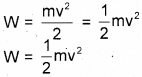Work is equal to the magnitude of kinetic energy
i.e, Kinetic energy K = $$\frac { 1 }{ 2 }$$ mv2,
M – mass, V – Velocity
When a body of mass m moves with a velocity v, its kinetic energy will be K = $$\frac { 1 }{ 2 }$$ mv2

Question 21.
A man having a mass of 70kg is riding a scooter of mass 80kg. What is the total kinetic energy if the velocity of the scooter is 10m/s?
m = 70kg + 80kg = 150kg
v = 10m/sQuestion 22.
A car of mass 1500 kg is moving at a velocity of 20m/s. What is its kinetic energy?
m = 1500 kg
v = 20m/s
K = $$\frac { 1 }{ 2 }$$ mv2
= 1/2 × 1500 × 202
= 300000J = 300kJ

Question 23.
A boy of mass 50kg is riding a bicycle with a speed 2m/s. The bicycle has a mass of 10kg. Calculate the total kinetic energy?
m = 50kg + 10kg = 60kg
v = 2m/s
K = $$\frac { 1 }{ 2 }$$ mv2
1/2 × 60 × 22 = 120J

Potential EnergyWhen we lift a body in perpendicular direction, the work is said to be done against gravitational force. According to figure, maximum work is done when it reaches at a height fb from the group, That means as the height increases work done also increases.

• The energy received by the body is equal to the work done on it.
• The body attains more energy when the height from the ground level increase

The energy possessed by a body due to its position is the potential energy.
ie, Potential energy
U = mgh
m – mass, g – acceleration due to gravity
h = height from the ground

Question 24.
Identify more situation in which potential energy is acquired by virtue of position

• Coconut in a coconut tree
• Water stores in huge reservoirs
• Objects placed above the buildings,

Inference:
Height increases, potential energy increases

Question 25.
Write down situations in which potential energy varies.

• Falling of a coconut from a coconut tree
• Pumping water to a tank at a height

Question 26.
Calculate the potential energy of a body of mass 1 kg at a height of 6m from the ground?
Mass m = 1 kg, Acceleration due to gravity
g = 10m/s2
h = 6 m,
U = mgh = 1 × 10 × 6 = 60J

Question 27.
A bird of mass 0.5 kg is flying at the same speed at the same height of 5m. In this state, if its Kinetic energy and potential energy are equal.
a) What is the potential energy of the bird?
b) What is the velocity of the bird?
a) m = 0.5kg
g = 10m/s2
h = 5m
u = mgh
= 0.5 × 10 × 5 = 25J
b) Kinetic energy K = 25J (∴U = K)Question 28.
Write other examples for getting potential energy due to strain• A Stretched bow
• An elongated rubber band
• Compressed spring
• Compressed spring in a toy car

Conclusion: The factor which gives potential energy are position and strain

Law Of Conservation Of EnergyQuestion 29.
What form of energy does the flower pot have when it is on the sunshade of a building?
Potential energy

Question 30.
While the flower pot is falling down, what forms of energy does it possess?
potential energy and kinetic energy

Question 31.
Does its potential energy increase/decrease when the pot falls down.
Potential energy decreases

Question 31.
Will the kinetic energy increase/decrease at that time?
Kinetic energy increases

Question 33.
What energy transformation takes place just before the flower pot reaches the ground?
Potential energy is converted completely into kinetic energy

Question 34.
Let the mass of the flower pot 15kg and the height of the sunshade 4m.
a) When the flower pot is on the sunshade, what is its potential energy? (g = 10m/s2).
b) When it is on the sunshade, what is its kinetic energy?
c) If so, what is its total energy?
U = mgh
= 15 × 10 × 4
= 600J
b) Kinetic energy will be zero
c) Total energy = PE + KE
= 600J + 0 = 600J

Question 35.
While falling, when the flower pot is at a height of 2m from the ground, what will be its kinetic energy?
K = $$\frac { 1 }{ 2 }$$ mv2
u = 0, g = 10m/s2 ,
s = 4 – 2 = 2m
v2 = u22 + 2as
=0 + 2 × 10 × 2 = 40
K = 1/2 × 15 × 40
= 300J

Question 36.
What is the potential energy when it is at a height of 2m from the ground? What is the total energy now?
Potential energy at 2m height
U = mgh = 15 × 10 × 2 = 300J
∴ Total energy = 300 + 300 = 600J

Question 37.
What is the kinetic energy of the flower pot just before it touches the ground?
K = $$\frac { 1 }{ 2 }$$ mv2
v2 = u2 + 2as
= 0 + 2 x 10 x 4 = 80
Kinetic energy K = $$\frac { 1 }{ 2 }$$ mv2
= 1/2 × 15 × 80 = 600J

Question 38.
The potential energy
U = mgh
= 15 x 10 x 10 x = 0.
What will be the total energy?
Total energy = 600 + 0 = 600J

Question 39.
To sum up the amount of energy at each situation:
b) When at a height of 2m from the ground
c) Just before hitting the ground
a) When on the sunshade = 600J
b) At a height of 2m from the ground = 600J
c) Just before hitting the ground = 600J
Energy can neither be created nor be destroyed. It can be transformed from one from to another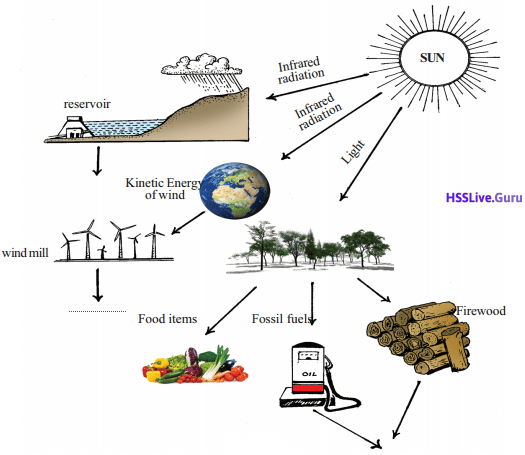Sun is the major source of energy. We utilize solar energy in different ways. It is renewable source of energy Plants prepare food by using sunlight. From these fossil fuels, firewood are formed. Windmills works by utilizing kinetic energy of wind energy from infra¬red rays. Tidal energy is obtained due to gravitation. When we consider any energy sources, they were originated from solar energy.

Power

Question 40.
Given below is the information regarding the working of pumps in three neighboring houses. Complete the table (g = 10 m/s2).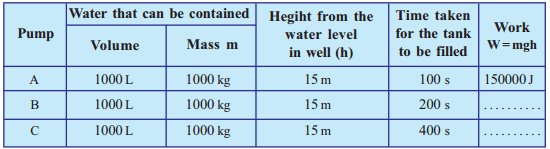b) 150000J
c) 150000J

Question 41.
Is the amount of work done by the pump to fill water in the three tanks equal?
Amount of work done is equal

Question 42.
Find the amount of work done per second by each pump.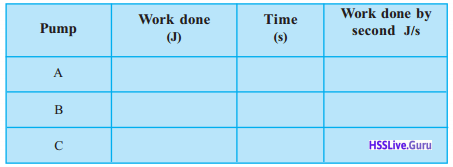Pump Work done Time (s) (J) Work done per second (J/S) A 150000 100 1500 B 150000 200 750 C 150000 400 375

Amount of work done per second is referred as power of the pump.
Work done per unit time or rate of doing work is power
Power = $$\frac{\text { work }}{\text { time }}, P=\frac{w}{t}$$
Units of power
watt (W), kilowatt (KW), Horse power (H.P)
1 kW= 1000W
1 HP = 746W

Question 43.
If a man of mass 70kg climbs up a mountain of height 30m in 5 minutes, what is his power?
m = 70kg, g = 10m/s2
h = 30m
Work W = mgh = 70 × 10 × 30 = 21000J
Time t = 5mt = 5 × 60 = 300s
Power p = $$\frac { w }{ t }$$ = $$\frac { 21000 }{ 300 }$$ = 70W

Question 44.
If a man of mass 50kg takes 60s to climb up 20 steps, each 15cm high, calculate his power.
m = 50kg, g = 10m/s2
h = 15cm × 20 = 300cm = 8m
t = 60s
work W = mgh = 50 × 10 × 3 = 1500J
Power p = $$\frac { w }{ t }$$ = $$\frac { 1500 }{ 60 }$$ = 25W

Let Us Assess

Question 1.
A boy is trying to push the concrete pillar of the building using a force of 300N. Calculate the amount of work done by the boy.
F= 300N, s = 0
w = Fs = 300 × 0 = 0

Question 2.
From what you have learnt of potential energy and kinetic energy write down the from of energy possessed by the bodies given below.
a) water in a dam
b) Stretched rubber band
c) Mango falling from a tree
a) Potential energy
b) Potential energy
c) Potential energy lesser. Kinetic energy greater

Question 3.
Calculate the kinetic energy of an athlete of mass 60kg running with a velocity 10 m/s
m = 60kg
v= 10m/s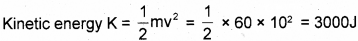Question 4.
A stone of mass 2kg is thrown upwards from the ground with a velocity of 3m/s. When it reaches maximum height, calculate its potential energy.
u = 3m/s v = 0
a = g = -10m/s2 (Velocity of a body moving upwards is decreasing so acceleration is negative)
v2 = u2 + 2as
0 = 32 + 2 × -10 × s
0 = 9 – 20s
20s = 9, s = $$\frac { 9 }{ 20 }$$
U = mgh = 20 × 10 × $$\frac { 9 }{ 20 }$$ = 90J

Question 5.
The heart of a healthy person beats 72 times per minute and each beat uses up about 1J of energy. Calculate the power of the heart.
W= 1 x 72 = 72J
t = 1mt = 60s
P = $$\frac { W }{ t }$$ = $$\frac { 72 }{ 60 }$$ = 1.2w

Question 6.
Which among the following is a vector quantity?
a) work
b) momentum
c) power
d) energy
momentum

Question 7.
If the velocity of an object is doubled, its kinetic energy becomes
a) 2 times
b ) 1/2
c) 4 times
e) 1/4
4 times

Question 8.
An object of mass 1 kg is falling from a height 10 m. What be the work done while falling?
a) 10 J
b) 1J
c) 100 J
d)1000 J
w = mgh = 1 × 10 × 10 = 100J

Question 9.
Which one among the following is correct?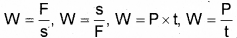W = p × t

Question 10.
A roller weighing 1 tonne is being dragged along a road. What is the work done against gravity? Why?
Zero, No displacement in the direction of force.

Question 11.
Is it possible for an object to possess energy without momentum? Give one example for such a situation.
Yes, possible. A coconut on a coconut tree has potential energy, but it has no momentum.

Question 12.
Say whether the following are positive work or negative work.
1) The work done by a person drawing water from a well using a rope without a pulley.
2) Work done by gravitational force in this situation
3) Work done by the frictional force while and object is sliding down along an inclined plane.
4) Work done by the force is moving along a plane surface.

1. Positive work
2. Negative work
3. Negative work
4. Positive work

Question 13.
How much joule is 1 kWh?
1 kWh = 1000 × 60 × 60 = 3600000J

Question 14.
Find out the work done against the gravitational force in the situations given below.
1. A child is standing still with a bundle of books of mass 5 kg
2. With the same bundle of books, she travels 1m along a plane surface with a speed 5 m/s
3. The bundle of books is lifted onto the top of a cupboard having 1m height (g=10m/s2).
1. 0
2. 0
3. W = mg = 5 × 10 × 1 = 50 J

Question 15.
A ball of mass 0.4 kg is thrown vertically upward with a velocity 14 m/s. Calculate its kinetic energy and potential energy after 1 s.
(Hint: v + at, s = ut + 1/2at2)
v = u + at
= 14 + 10 × 1
= 24 m/s
KE = $$\frac { 1 }{ 2 }$$ mv2
= 1/2 × 0.4 × 24
= 115.2 J
S = ut + 1/2 at2
= 14 × 1 + 1/2 × 10 × 12
= 14 + 5 = 19 m
E = mgh = 0.4 × 10 × 19
= 76 J

Question 16.
An object of mass 1000 kg is travelling with a velocity 72 km/h. Calculate the work done to bring it rest.
m = 1000 kg
v = 72 km/h = 20 m/s
Work = difference of kinetic energy
= $$\frac { 1 }{ 2 }$$ mv2
= $$\frac { 1 }{ 2 }$$ × 1000 × 20 × 20 = 400000J

Question 17.
Estimate the work done on a object of mass 80 kg to change its velocity from 5 m/s to 10 m/s
m = 80 kg
Work = difference of kinetic energy### Work, Energy and Power More Questions

Question 1.
Fill in the blanks
a) Work done when a body of mass 100g is lifted up to a height of 1 meter is ……….
b) The two factors related to potential energy to body are………….
c) 1HP = ……… Watt
1 Joule
b) Position, Strain
c) 746W

Question 2.
Classify the following into work done and work is not done
1. A mango falling from a mango tree
2. Pushing a table while sitting on it
3. Pushing a wall
4. Kicking a football
5. A trolley is moving forward
Work done:
1. A mango falling from a mango tree
2. Kicking a football
3. A trolling is moving forward
Work is not done:
1. Pushing a table while sitting on it
2. Pushing a wall

Question 3.
When Lekshmi is applied a force of 50N on an object it undergoes a displacement of 2m. When Vinitha applied the same force on itundergoesadisplacementof3m if so
a) Which person done more work?
b) Give reason
c) Calculate the work done by each person?
a) Vinitha
b) Displacement is greater
c) Work done by Lekshmi
W = Fs = 50N × 2m = 100J
Work done by Vinitha
W = Fs = 50N × 3m = 150J

Question 4.
Calculate work done when a boy of mass 40kg climb¬ing a staircase of height 50cm, g = 10m/s2
W= Fs
F = mg = 40 × 10 = 400N
S = h = 0.5m
W= 400 × 0.5 = 200J

Question 5.
a) What is mean by kinetic energy?
b) How mass and velocity affect kinetic energy?
c) A body of mass 20kg is moving with a velocity of 5m/s. Calculate the kinetic energy?
a) The energy possessed by a body by virtue of its motion is called kinetic energy
b) Mass increases, kinetic energy increases mass . is doubled kinetic energy also doubled. Velocity increases, kinetic energy increases, Velocity is doubled kinetic energy becomes four times.
c) Kinetic energy KE = $$\frac { 1 }{ 2 }$$ mv2
= 1/2 × 20 × 52 = 1/2 × 20 × 25 = 250J

Question 6.
a) Write the equation for finding potential energy?
b) indicate the representation of each letter
a) U = mgh
b) M – mass,
g – acceleration
h – height from the ground

Question 7.
State law of conservation of energy?
Energy can neither be created nor be destroyed. It can be transformed from one form to another.

Question 8.
A store of mass 5kg was raised from the ground to the second floor of height 7m and from there to the third floor of height 3m from the second floor. Calcu¬late the potential energy of the store with respect to the ground floor and the second floor?
Mass m = 5kg
Height from ground to third floor
h = 7m + 3m
g = 10m/s2
Potential energy with respect to the ground
U = mgh = 5kg × 10m/s2 × 10 = 500J
Height from second floor to third floor h = 3m
Potential energy with respect to the second Floor
U = mgh = 5kg × 10m/s × 3 = 150J

Question 9.
a) What is power?
b) The time takes to move an object at a distance of 5m is 10s is the force applied is 30N, what is the power?
Power P = $$\frac { 150 }{ 10s }$$ = 15W